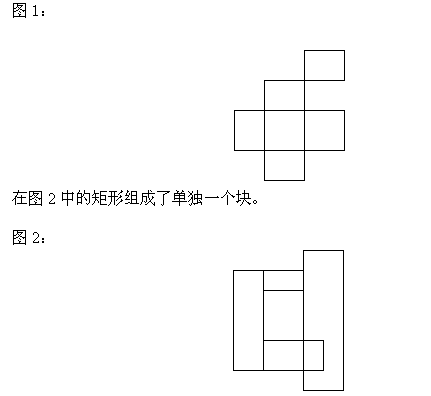# 矩形 题解

### 题目### 代码

#include<iostream>
#include<cstdio>
using namespace std;
struct hhx{
int x1,y1,x2,y2;
}h;
int n,xx,yy,ans,f;
int find(int x)  //查找父节点
{
if (f[x]==x) return x;
return f[x]=find(f[x]);
}
bool pd(hhx t,hhx x)
{
if (t.x1>x.x2||x.x1>t.x2||t.y1>x.y2||x.y1>t.y2)
return false;
else if ((t.x1==x.x2||t.x2==x.x1)&&(x.y1==t.y2|x.y2==t.y1))
return false;
else return true;
}
int main()
{
scanf("%d",&n);
for (int i=1;i<=n;i++)
f[i]=i;
for (int i=1;i<=n;i++)
{
scanf("%d%d%d%d",&h[i].x1,&h[i].y1,&h[i].x2,&h[i].y2);
for (int j=1;j<i;j++)
{
xx=find(i),yy=find(j);
if (pd(h[i],h[j])&&xx!=yy)  //有连接
f[xx]=yy;  //合并
}
}
for (int i=1;i<=n;i++)
if (f[i]==i)
ans++;
cout<<ans<<endl;
return 0;
}

06-2792
10-1243111-10247
03-1267
01-20391
11-17473
02-1431万+
04-07746
09-265万+
07-2543